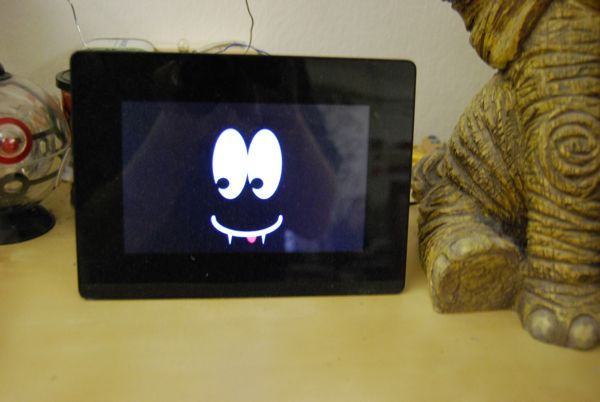## Little Picture frame turned into a Vampire

Back in march I made a small processing sketch that generates eyes for a cheep digital picture frame ( see Little Pictureframe is watching you),

this little picture frame has turned into a vampire todayHere is the processing sketch I used to generate the images. If you don't want to generate your own picturs here is a zip containing some example picture

``````void setup() {
size(768,480);
smooth();
}

float d = random(360);
float e = random(150) + 15;

void draw() {
background(0);
noStroke();

// eyes
fill(255);
ellipse(334, 180, 100, 200);
ellipse(434, 180, 100, 200);
fill(0);

float a = radians( d );

ellipse(334 + cos(a) * 30, 180 + sin(a)*80, 40, 40);
ellipse(434 + cos(a) * 30, 180 + sin(a)*80, 40, 40);

// tongue
pushMatrix();
translate( 384, 340 );
float c = radians( e );
translate( sin( c - PI/2 ) * 100, cos( c - PI/2 ) * 50 );
rotate( -c + PI/2 );
noStroke();
fill( 255,0,0);
arc( 0, 0, 30, 50, 0, PI );
popMatrix();

// mouth
stroke(255);
strokeWeight(10);
noFill();
arc( 384, 340, 200, 100, 0, PI );

// tooth
fill(255);
noStroke();
beginShape();
vertex(328,385);
vertex(334,420);
vertex(340,385);
endShape(CLOSE);

beginShape();
vertex(428,385);
vertex(434,420);
vertex(440,385);
endShape(CLOSE);

}

void keyPressed() {
d = random(360);
e = random(150) + 15;
redraw();
saveFrame();
}
``````